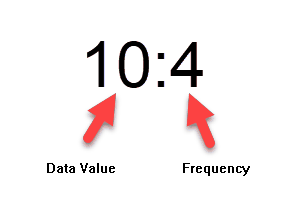﻿ Frequency Tables

# Frequency Tables

Top  Previous  Next

You can include frequency information in data parameters using the following format:You can list these points out in a data parameter, with the values separated by commas (or semicolons if you use a comma as a decimal point)

\$f=data(10:2,11:5,12:4,13,14:2,15:2)

This is equivalent to this frequency table.

 x f 10 11 12 13 14 15 2 5 4 1 2 2

All statistical commands (mean, median, standard deviation...) will utilize the frequency information when calculating their values.

You can access individual entries in the data parameter using references. For example, if you type \$f, the system will return 12:4.

You can access the data value and frequency components of the table using the datavalue and freq commands in the calculator.

{datavalue(\$f)}        will return 12

{freq(\$f)}                will return 4